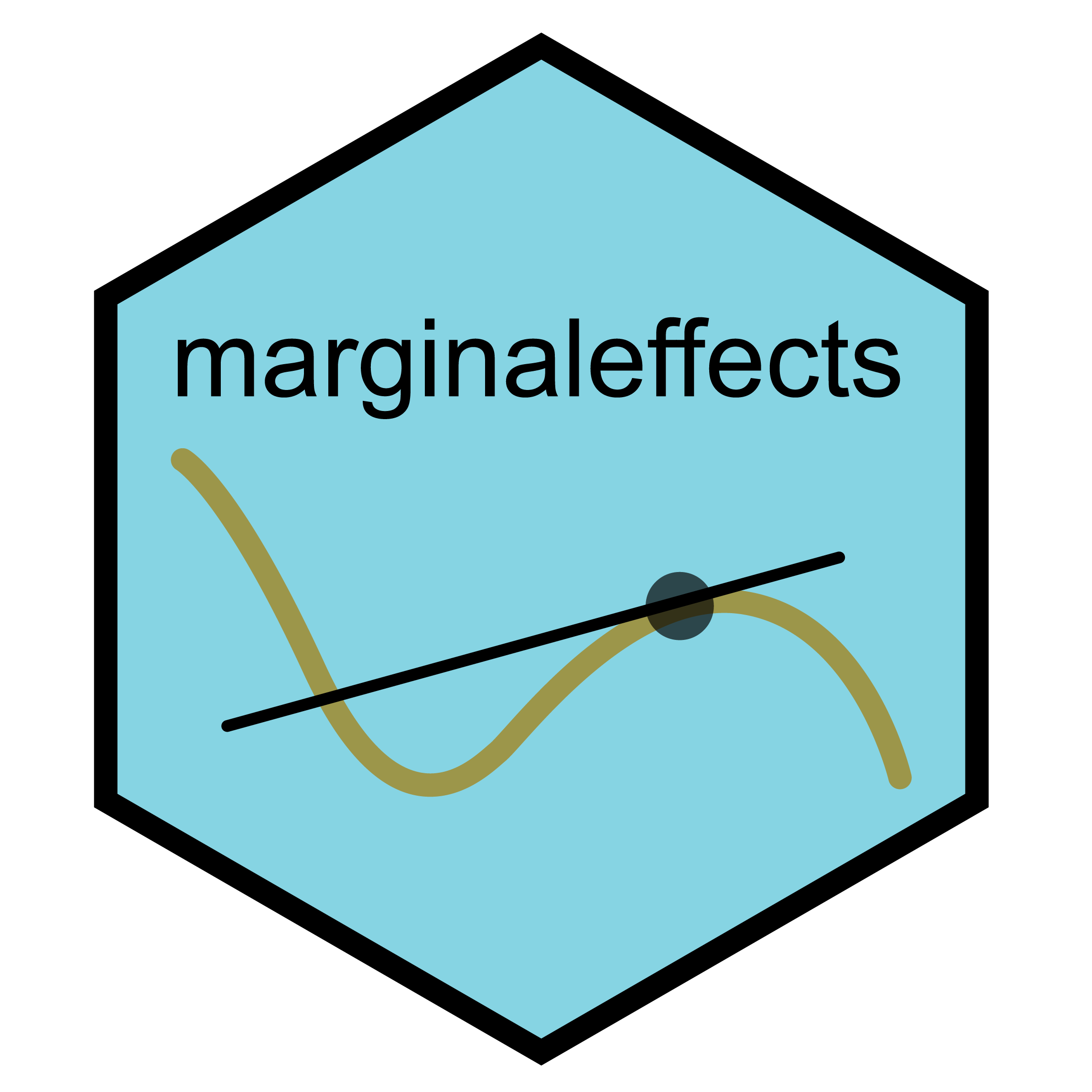# The Marginal Effects Zoo (0.15.1)

Author

Vincent Arel-Bundock🚨🚨🚨 This November, the `marginaleffects` author (Vincent) will be giving an online seminar called: “Interpreting and Communicating Statistical Results with R.” If you want to become a `marginaleffects` expert and support the development of the package, sign up at Code Horizons! 🚨🚨🚨

# 1 Why?

Interpreting the parameters estimated by complex statistical models is often challenging. Many applied researchers are keen to report simple quantities that carry clear scientific meaning but, in doing so, they face three primary obstacles:

1. Intuitive estimands—and their standard errors—are often tedious to compute.
2. The terminology to describe these estimands is not standardized, and varies tremendously across disciplines.
3. Modeling packages in `R` and `Python` produce inconsistent objects which require users to write custom (and error-prone) code to interpret statistical results.

The “Marginal Effects Zoo” book and the `marginaleffects` packages for `R` and `Python` are designed to help analysts overcome these challenges. The free online book provides a unified framework to describe and compute a wide range of estimands. The `marginaleffects` package implements this framework and offers a consistent interface to interpret the estimates from over 86 classes of statistical models.

# 2 What?

The `marginaleffects` package allows `R` and `Python` users to compute and plot three principal quantities of interest: (1) predictions, (2) comparisons (contrasts, risk ratios, odds, lift, etc.), and (3) slopes. In addition, the package includes a convenience function to compute a fourth estimand, “marginal means”, which is a special case of averaged predictions. `marginaleffects` can also average (or “marginalize”) unit-level (or “conditional”) estimates of all those quantities. Finally, `marginaleffects` can also conduct hypothesis and eequivalence tests on coefficient estimates and on any of the quantities generated by the package.

Predictions:

The outcome predicted by a fitted model on a specified scale for a given combination of values of the predictor variables, such as their observed values, their means, or factor levels. a.k.a. Fitted values, adjusted predictions. `predictions()`, `avg_predictions()`, `plot_predictions()`.

Comparisons:

Compare the predictions made by a model for different regressor values (e.g., college graduates vs. others): contrasts, differences, risk ratios, odds, lift, etc. `comparisons()`, `avg_comparisons()`, `plot_comparisons()`.

Slopes:

Partial derivative of the regression equation with respect to a regressor of interest. a.k.a. Marginal effects, trends. `slopes()`, `avg_slopes()`, `plot_slopes()`.

Marginal Means:

Predictions of a model, averaged across a “reference grid” of categorical predictors. `marginalmeans()`.

Hypothesis and Equivalence Tests:

Hypothesis and equivalence tests can be conducted on linear or non-linear functions of model coefficients, or on any of the quantities computed by the `marginaleffects` packages (predictions, slopes, comparisons, marginal means, etc.). Uncertainy estimates can be obtained via the delta method (with or without robust standard errors), bootstrap, or simulation.

# 3 Benefits

The advantages of `marginaleffects` include:

• Powerful: It can compute predictions, comparisons (contrasts, risk ratios, etc.), slopes, and conduct hypothesis tests for 86 different classes of models in `R` and `Python`.
• Simple: All functions share a simple and unified interface.
• Documented: Each function is thoroughly documented with abundant examples. The website includes 20,000+ words of vignettes and case studies.
• Efficient: Some operations are orders of magnitude faster than with the `margins` package, and the memory footprint is much smaller.
• Thin: Few dependencies.
• Standards-compliant: `marginaleffects` follows “tidy” principles and returns objects that work with standard functions like `summary()`, `head()`, `tidy()`, and `glance()`. These objects are easy to program with and feed to other packages like `modelsummary`.
• Valid: When possible, numerical results are checked against alternative software like `Stata` or other `R` packages. Unfortunately, it is not possible to test every model type, so users are still strongly encouraged to cross-check their results.
• Extensible: Adding support for new models is very easy, often requiring less than 10 lines of new code. Please submit feature requests on Github.
• Active development: Bugs are fixed promptly.

The `marginaleffects` package is licensed under the GNU General Public License v3.0. The content of this website/book is licensed under the Creative Commons Attribution-NonCommercial 4.0 International (CC BY-NC 4.0).

``````Warning in citation("marginaleffects"): no date field in DESCRIPTION file of package 'marginaleffects'

To cite package 'marginaleffects' in publications use:

Arel-Bundock V (2023). _marginaleffects: Predictions, Comparisons, Slopes, Marginal Means, and Hypothesis Tests_. R package version 0.15.1, <https://marginaleffects.com/>.

A BibTeX entry for LaTeX users is

@Manual{,
title = {marginaleffects: Predictions, Comparisons, Slopes, Marginal Means, and Hypothesis
Tests},
author = {Vincent Arel-Bundock},
year = {2023},
note = {R package version 0.15.1},
url = {https://marginaleffects.com/},
}``````